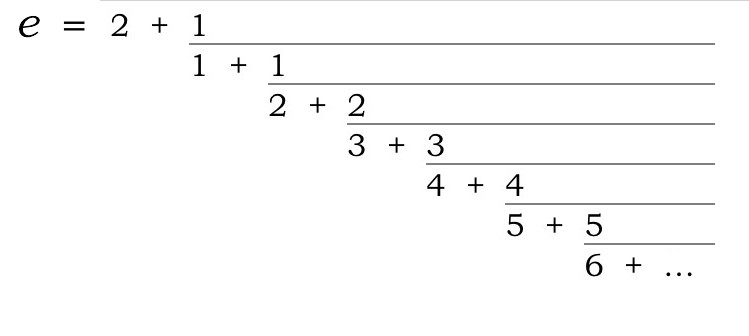☜ Navigate through the Golden Ratio pages ☞

# Continued Fraction Calculator

Automated calculation of continued fractions for (simple) expressions or decimal numbers.

## Brief Explanation

A continued fraction is a kind of fraction that is not reduced. Now, normally you are taught to reduce fractions to their simplest forms. But you can go the other way, and expand them, and what comes out can show you something about the number. Continued fractions (CFs for short) are useful in finding rational approximations to irrational numbers.

It is that very aspect of CFs that sheds light on the golden ratio φ.

## Try It Yourself

To find the CF for a value or expression, just type it into the text field with the blue border and hit the tab or return key. A decimal number like “1.5” will work, as will a fraction like “3/7”. Or use one of the buttons below to see some interesting CFs.

For advanced users: note that unless you have just one expression, like “exp(3)”, you must use JavaScript notation. For example, “Math.exp(3) + Math.cos(5)”. If there is only one expression, the “Math.” is automatically prepended.
Expression for xDecimal expansion of x
=

Continued fraction for x in short form

Continued fraction in long form

### Interesting values to try

• x = As a decimal it repeats, but the continued fraction stops.
• x = Everyone’s favorite constant. Or should it be ?
• x = Base of the natural logarithm. As a decimal, it does not repeat, but the continued fraction has a clear pattern!
• x = Another irrational number with a pattern.
• x = How does this differ from the previous example?
• x = This is our key example: Phi, the golden ratio.
• x = The Feigenbaum constant.
• x = An obscure one, ii. Convince yourself that it is the same as e-π/2.

## Remarks

It may look mysterious, but finding a CF is quite simple. Take the familiar example of π. Rewrite it as

π = 3 + .14159…

Now, find the reciprocal of the fractional part, that is, 1/.14159… = 7.0625… (Note that the reciprocal is guaranteed to be greater than 1, so there will always be an integer part to pluck, like a red cherry.)

So now we have

π = 3 + 1/7.0625…

Now, repeat the procedure with the 7.0625… part:

π = 3 + 1/(7 + .0625…)

and find the reciprocal of the fractional part, that is 1/.0625… = 15.996…, so:

π = 3 + 1/(7 + 1/15.996…)

Repeating this process gives the CF.

If a number is rational, then the CF is finite. But, as the above example of 3/7 shows, even though the CF is finite, the decimal is not. So in some ways, the CF is more convenient than the repeating, non-terminating decimal.

If a number is irrational, then both its decimal and its CF are infinitely long. But although there will (usually ) be no pattern in the decimal, there may be a pattern in the CF. The examples of e and φ show this clearly. If there is a good explanation of the pattern for e, I have never seen it.

Interestingly, if we relax the canonical-form constraint that all the numerators must be 1, there is another very patterned continued fraction for e, discovered by Euler:Surely there is an intuitive derivation of e that uses this CF to improve on the “compound interest” rationale. Which never seemed that intuitive to me.

The next page will discuss what we can learn about φ from its particularly simple CF.

The term “pattern” is of course very general. For example, the number 1.01001000100001… is irrational but has a distinct pattern. (Hence we are justified in using the ellipsis … to imply that you get the pattern.) But there is no single block of digits that repeats.

☜ Navigate through the Golden Ratio pages ☞